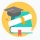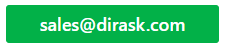Home
IT Knowledge
IT Career
Inspiration
Languages
EN

# Java - insert node as first one to double linked list (custom implementation)

6 points
Created by:AnnLen
9180

## 1. Overview

In this post we will see how to implement algorithm to correctly add node as first one to double linked list. Below we have 2 implementations, first one with node based on Integer, second one with generic Node and usage example based on String. To create new Node we call constructor with previous node set as null and next node set as newly created one.Then we have short logic which will handle linking our nodes together. We can use the logic to reverse the process of adding the node as first one and implement logic for appending the node as the last one. In next article we cover the algorithm of inserting node to the end of linked list (adding node as last one).

## 2. Insert node - code example

Below we have java implementation with Node based on Integer as item of double linked list with logic to insert node as first one. This implementation is without generics to have this example as simple as possible. Generic implementation of this linked list is in next part of this post.

``````package com.dirask;

Node first;
Node last;
int size;

Node tmpFirst = first;
Node newNode = new Node(null, item, tmpFirst);
first = newNode; // assign new node as fist
if (tmpFirst == null) {
last = newNode;
} else {
// take care that each node has previous link
tmpFirst.prev = newNode;
}
size++;
}

@Override
public String toString() {
if (first != null) {
return first.printForward();
}
return "List is empty";
}

private static class Node {
Node prev;
Integer item;
Node next;

public Node(Node prev, Integer item, Node next) {
this.prev = prev;
this.item = item;
this.next = next;
}

public String printForward() {
if (next != null) {
return item + " -> " + next.printForward();
}
return String.valueOf(item);
}
}

public static void main(String[] args) {

// list: 3 -> 2 -> 1
System.out.println("list: " + list.toString());
// size: 3
System.out.println("size: " + list.size);
}
}
``````

Output:

``````list: 3 -> 2 -> 1
size: 3``````

## 3. Insert node - generic implementation

Below we have java generic implementation of double linked list with add first method which will add node to our list.

``````package com.dirask;

Node<E> first;
Node<E> last;
int size;

Node<E> tmpFirst = first;
Node<E> newNode = new Node<>(null, item, tmpFirst);
first = newNode; // assign new node as fist
if (tmpFirst == null) {
last = newNode;
} else {
// take care that each node has previous link
tmpFirst.prev = newNode;
}
size++;
}

@Override
public String toString() {
if (first != null) {
return first.printForward();
}
return "List is empty";
}

private static class Node<E> {
Node<E> prev;
E item;
Node<E> next;

public Node(Node<E> prev, E item, Node<E> next) {
this.prev = prev;
this.item = item;
this.next = next;
}

public String printForward() {
if (next != null) {
return item + " -> " + next.printForward();
}
return String.valueOf(item);
}
}

public static void main(String[] args) {

// list: 3 -> 2 -> 1
System.out.println("list: " + list.toString());
// size: 3
System.out.println("size: " + list.size);
}
}``````

Output:

``````list: 3 -> 2 -> 1
size: 3``````

## References (Harvard CS classes - Data Structures)